Categories

## Springer Books

Yesterday I noticed on Twitter that Springer have made available quite a few classic books from their “Undergraduate Texts in Mathematics” and “Graduate Texts in Mathematics” freely available as PDFs. Some of these I paid good money for as an undergraduate and still have on my shelves and dip into occasionally.

A GitHub user “bishboria” has usefully made a hyperlinked list of all the books from these series that are available for free as a GitHub Gist here. If you are so inclined further down the page are shell scripts that you can run to automatically download all of them.

There is a great variety of books available, but if you only want to download one, in my opinion a book that is definitely worth having is Donald Estep’s “Practical Analysis in One Variable”. This is an amazing book that covers topics ranging from the natural numbers and the invention of negative numbers up to topics in analysis such as Weierstrass’ approximation theorem. Donald Estep is also interesting to follow on Twitter at @donestep1. As an aside he is also one of the authors of the books for the “Applied Mathematics: Body and Soul” project.

Categories

## New AS-Level Further Mathematics 2017

Following on from Stuart’s (@sxpmaths) excellent blog post looking at the changes coming to A-Level mathematics in 2017 I thought I would take a bit more of a detailed look at the prescribed content of Further Mathematics than I did in my old post here. Since I wrote my original post there have been a few changes (notably clarification of how the prescribed content for AS-Level is decided) between the draft content and the finalised content document that was published in December 2014..

This post is my interpretation of the content contained in the content document  and I would encourage everyone to look at the original!

Unlike the 100% prescribed core of the standard A-Level only 50% of the content for Further Maths (FM) is prescribed with awarding bodies able to determine the other 50%. I’m not sure how I feel about this: part of me likes the freedom that this gives the exam boards to potentially include novel content and explore different areas of mathematics. The other part of me would prefer a 100% prescribed A-Level as this levels the playing field for students who (at the moment) are often at the mercy of their schools in terms of what they study to bolster results, not necessarily preparing them as well for future mathematical study. I can see this kind of “game playing” potentially occurring if there are vast differences between individual boards.

In this post I will look at the prescribed content of the AS-Level further mathematics qualification

AS-Level:

There is some prescribed core content for AS FM however a smaller proportion is prescribed than that for the full A-Level as explained in the original document.

• “At least 30% (approximately) of the content of any AS further mathematics specification must be taken from the prescribed core content of A level further mathematics.”

This 30% is composed of two parts. 20% of the overall content must come from the following areas

• Complex Numbers including:  the solution of quadratic, cubic and quartic equations (with real coefficients), arithmetic operations on complex numbers including the use of the complex conjugate. Students are to be able to represent complex numbers on an Argand diagram and know that complex roots of a polynomial (with real values coefficients) occur in complex conjugate pairs.  Manipulation of numbers in modulus argument form. Construction and interpretation of simple loci in the Argand diagram.
• Matrices including: Knowledge of the zero and identity matrices. Multiplication of a matrix by a scalar and addition, subtraction  and multiplication of conformable matrices. Use of matrices to represent single linear transformations in 3D (some restrictions apply) and successive linear transformations in 2D. Finding invariant lines and points of linear transformations. Determinants and inverses of $$2\times2$$ matrices.
• Further Algebra and Functions including: The relationship between the roots and coefficients of polynomial equations, up to quartic equations. Forming a polynomial whose roots are a linear transformation of the roots of a given polynomial equation, where the polynomial is at least of cubic degree.

Another (approximately) 10% of the overall specification must be taken from the rest of the prescribed content of A-Level FM, but it is up to the boards to decide what they take.

A few comments on the above

• When manipulating numbers in modulus-argument form knowledge of radians and compound angle formulae are assumed. Depending on how schools teach the AS this could prove problematic as radians are not introduced until A2.
• I like the inclusion of loci, in some boards this isn’t currently tackled until FP# and consequently may not be encountered by students at all.
• There seems to be more of a focus on finding invariants under linear transformations than at present.
• It’s nice to see the transformation of polynomials in there as it has wide ranging applications.

Essentially, only 20% of an AS qualification in further mathematics is necessarily common to all boards.

Come back early next week for my take on the rest of the prescribed content for the full A-Level further mathematics qualification.

Categories

## ATM MT #mathsjournalclub Special Edition Winning Articles

Apologies that this announcement is later than planned, but I still think there is time for people to read these articles for a discussion on Monday 11th January at 8pm.

The ATM Mathematics Teaching journal produced a special assessment focussed edition this November that has completely open access until the end of January and for this reason we decided to run an extra maths journal club chat looking at two articles from this issue.

The winning article was:

The second placed article was:

• “Assessment: Beyond right and wrong” by Matt Lewis – This is an account of an on-going evidence-based project in a number of schools in London. The professionals involved seek to set the complex process of assessment of learning in mathematics in the context of its educational and social purposes. The article draws on examples
from recent work on approaches to the assessment of reasoning and problem-solving. The challenges are not underestimated given that we seem to be experiencing a culture that might be regarded as valuing measurement of performance regardless of the quality or meaningfulness of the data generated. The debates will continue, but the enduring need for statutory assessment should not dumb-down the process.

If you click on the article titles you will be taken to a pdf file – both are fairly short and would make great Christmas reading.

I look forward to discussing these on the 11th January 2016 for the first #mathsjournalclub of the newyear.

Categories

## An App Review – SUMAZE!

This post is an expanded version of a review I wrote for the IMA’s (Institute of Mathematics and it’s Applications) magazine Mathematics Today which was published in the December 2015 issue. If you are in the UK ad aren’t already a member of the IMA I really would encourage you to join, the magazine is great and there are some great regional talks etc.

SUMAZE! is an app available for both Apple and Android devices and is a completely free download (and free of adverts).

I first became aware of MEI’s new mathematics game, SUMAZE!, at the beginning of half term after seeing it mentioned on Twitter. It is available for both iOS and Android devices and “awesome puzzle game” is typical of the reviews in these stores. Indeed, SUMAZE has already entered the top 10 educational apps chart in the Google play store despite only being released on the 20th October 2015. Sumaze is based on an idea of Richard Lissaman of MEI and was then developed into the apps we have today.

When you start the app you are presented with the following screen:At first the differing levels are locked and are opened as you complete more of them.

The game is simple in concept; you, as the player, just need to move a numbered block to an exit point. In working your way to the exit however, operator blocks modify the number on your block and green blocks only let you pass if your number satisfies the condition shown. The choice of operators and conditions can make all the difference in how quickly you can complete a level. The app is very well designed, with a slick look and an intuitive interface. Six core topics are covered by the currently available levels (arithmetic, negatives, powers, inequalities and modulus, logarithms and properties of number) and these are unlocked as you complete the levels. Whilst you may think that arithmetic is a simple mathematical concept, in fact some of the levels for each section can be surprisingly (and frustratingly) difficult! I was stuck on a particular level in the Numbers section for what felt like hours and one level in the final “Fermat’s Rooms” seemed very challenging. I like to think that there is a bug in this level concerning modular arithmetic.To me -1 should pass through the green condition block labelled “=1 mod 2” and then all the others down the right hand side. However this isn’t allowed and after forming some equations you can obtain a value in the 800s.

These mazes could be a great way for a teacher to introduce the concept of logarithms for example. Students working through the levels will develop a deeper conceptual understanding of what a logarithm “actually is” than they may have achieved through just the definition and some questions. It would be a help if there was a “teacher unlock” code to unlock levels on school devices so that particular levels could be used by the students.

The SUMAZE! puzzles also appear as resources on MEI’s integralmaths.org website for use in class. In addition, since writing the review for the IMA a web version has been made publicly available.\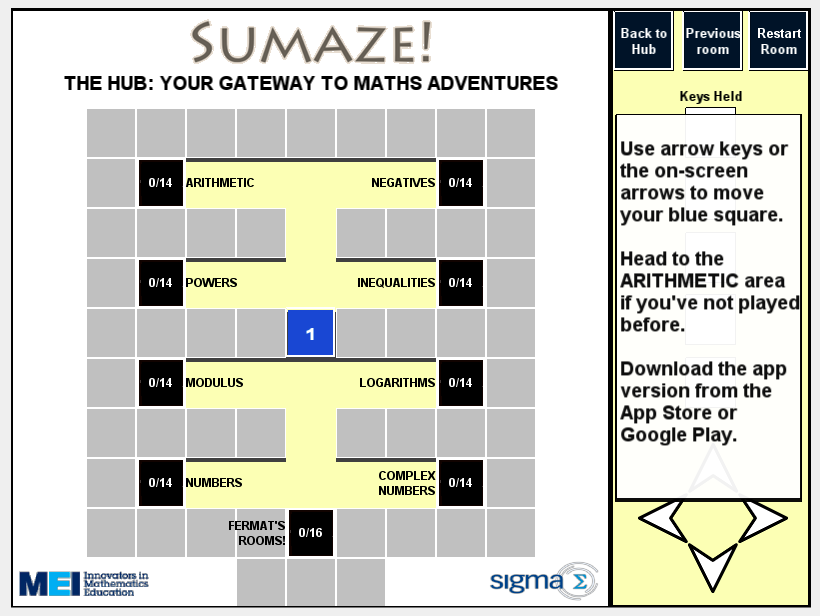In the web version all levels are unlocked and so can be used to introduce students to logarithms for example. I used this web app with a Year 7 class towards the end of the year, instructing them to do the arithmetic levels first and then allowed them to select between negatives, powers or modulus. A lot of them chose to try the modulus levels as this was something unfamiliar to them. It was really nice to watch them learn about the modulus function (a topic that they definitely wouldn’t normally come across at such an age) and be self motivated to do so.

I really hope that MEI produce some add-on levels for this game as I am now left wanting more! Similar apps for other topics would also be great, I can think of similar ideas that would be good for both younger and older target audiences.

As an aside, does anyone know who the “Dr H” some of the levels refer to is? I think I am missing some popular culture reference…..

I’ll end the review with a quote sent to me from Richard Lissaman that he had received from a user of the app

“It happened that I was visiting with my 15-year-old grand-daughter this afternoon and mentioned the Sumaze! app. This girl is something of a mathophobe, but no sooner had I mentioned the app than she downloaded it to her iPad. Then it was all that I could do to pry it away from her to talk about adding rational functions. Thanks so much for telling me about this”

If you haven’t already succumbed to this game, download it now, I promise that you will enjoy it.

Categories

## Wolfram Alpha and Precision of Input

This post mainly concerns the use of WolframAlpha and has been prompted by a tweet by Peter Rowlett on the 10th of December 2015.

WolframAlpha is an incredibly useful tool that more and more students are beginning to use to check their work (and, I’m sure in some cases just do the work for them!) and the free from input that is a large part of WolframAlpha’s mission makes it very easy to use.

However, sometimes this natural language free form output doesn’t result in what the user expects as this tweet from Peter (@peterrowlett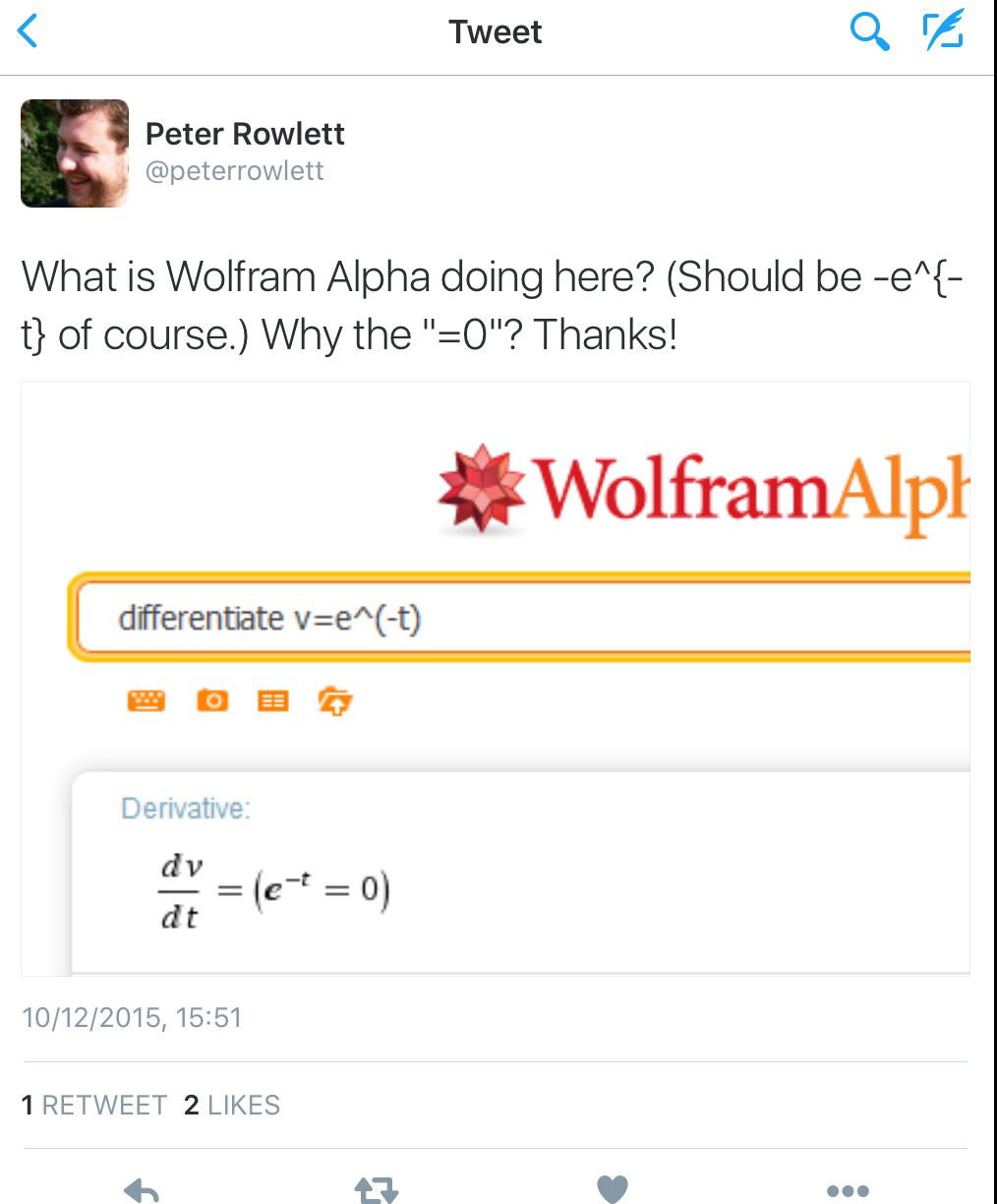Of course, we understand implicitly that $$v$$ is a function of $$t$$, but because WolframAlpha doesn’t know this it and differentiates both sides – taking $$v$$ to be a constant – and then multiplies by -1 on both sides (removing negative signs if possible is often seen as making an expression simpler). Stuart commented that mking $$v$$ a function of $$t$$ explicitly solves the problem.I then commented that I would normally just use the Wolfram Language / Mathematica syntax (D[v[t] = Exp[-t], {t,1}]) – of course this goes against the idea of using free form input but it does remove any ambiguity of what I am interested in WolframAlpha working out.

Ordinarily I use formal syntax almost all of the time when using WolframAlpha as I like the precision that it gives me, however yesterday I discovered that some commands don’t work correctly! for example, if I try to use the DSolve command to solve a second order differential equation with initial conditions I get an error: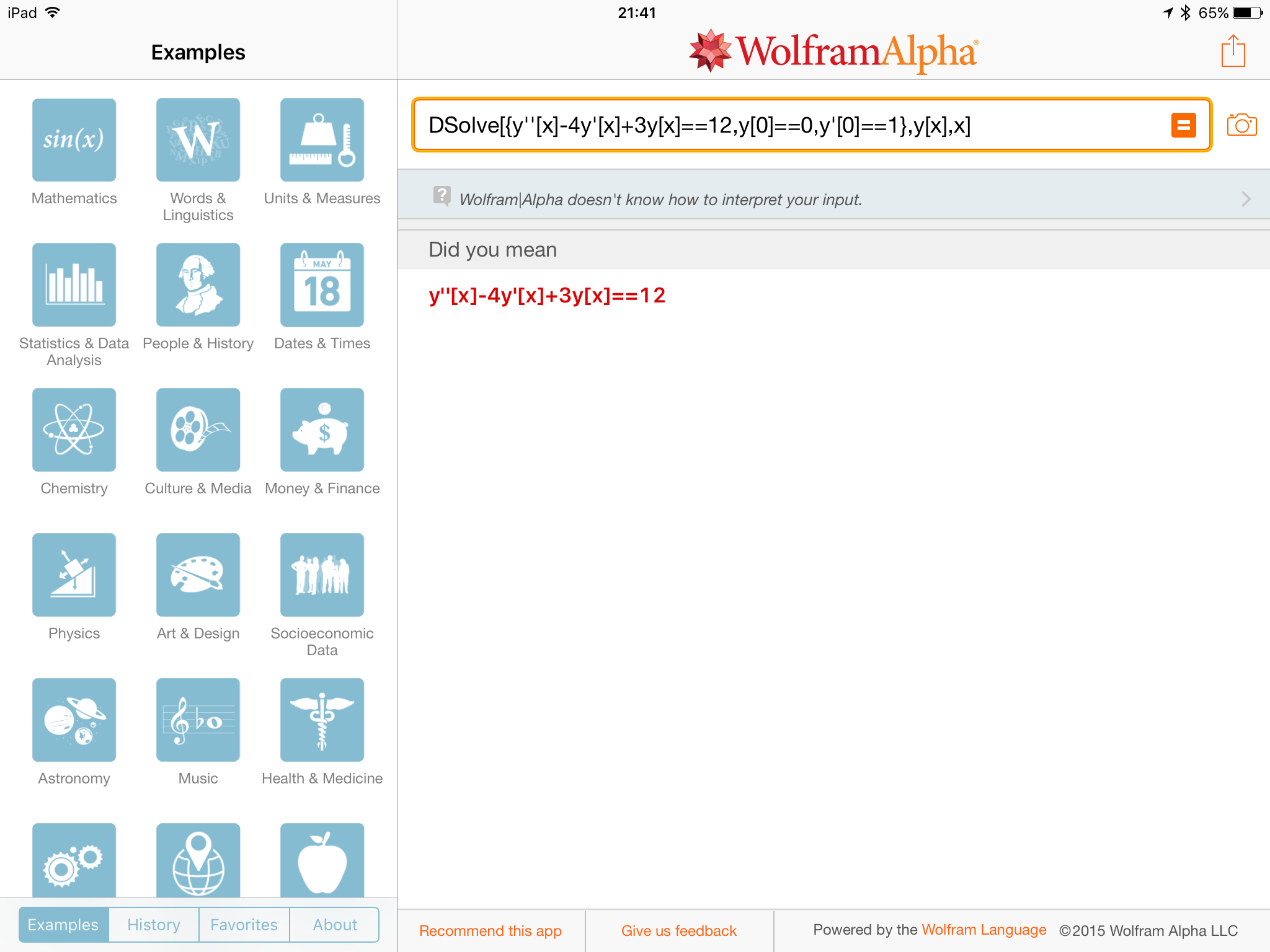whereas just typing the differential equation with initial conditions gives me the solution: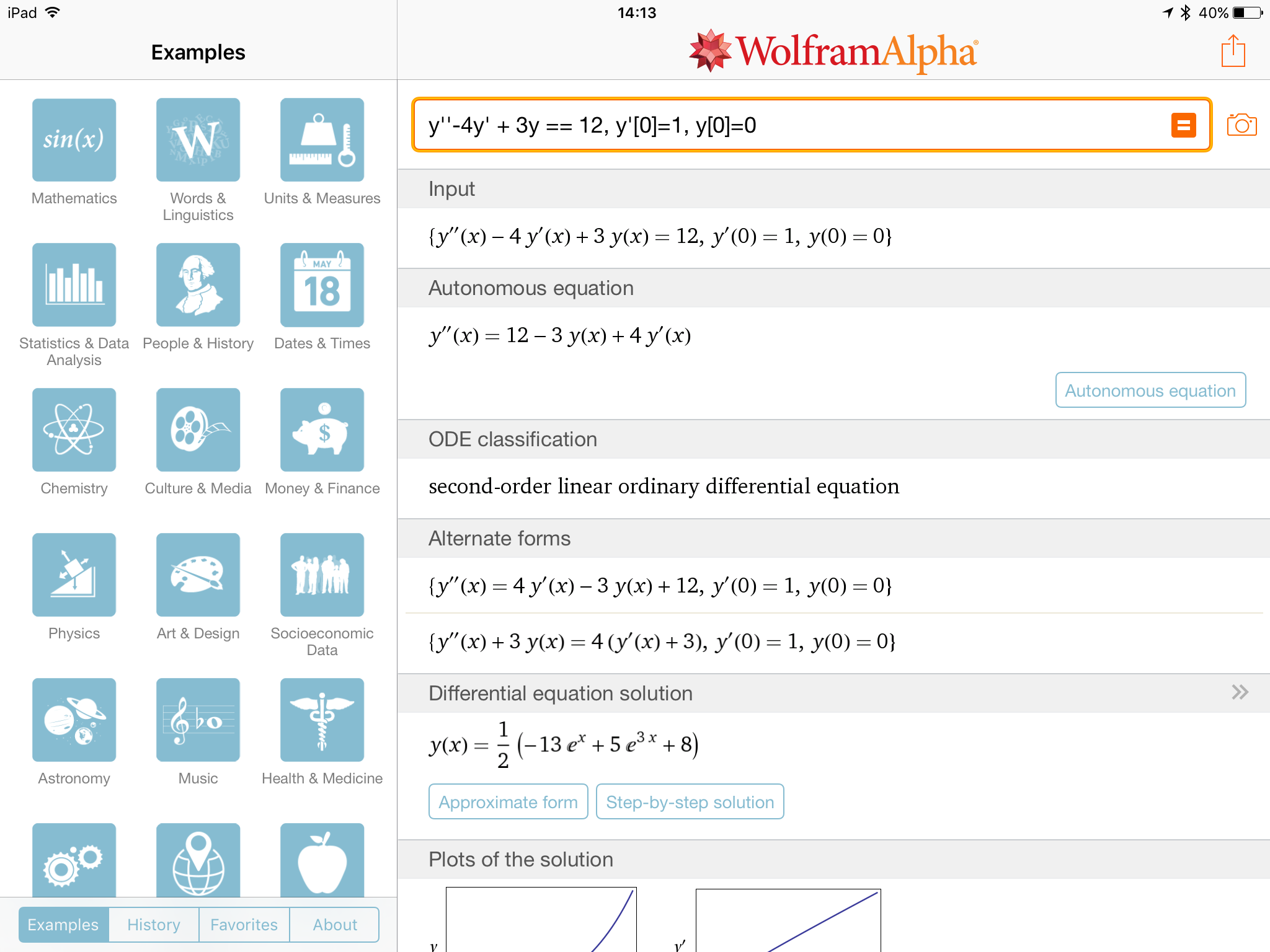I find this a bit odd – maybe Wolfram are trying to push free form input. Regardless, it is still important to be mathematically precise when entering functions to ensure that you get the correct result.

I’ve recently started using the WolramCloud for computations when I want to use formal syntax and I will blog about that some time soon!

Categories

## A Level Calculated Colouring – Corrections

Thank you for all the positive comments on the A-Level Calculated colouring resource that i shared earlier in the week, I’m glad that people have used it.

I’m extremely grateful to Adam Creen (@adamcreen), Ben Plowman (@PlowMaths) and Gary Wing (@gsw71) for pointing out a few questions that had typos or needed correcting. I have now corrected the documents linked in the original post, and below are what the corrected questions should be:

• Question 1 – “$$\int_0^2 2x+\frac{1}{2} \mathrm{d}x$$“
• Question 11 – “The greatest root of the equation $$x^2-3x-10 = 0$$.”
• Question 15 – “The value you obtain when you evaluate $$y = 3x^3-7x+7$$ at $$x = 1$$
• Question 18 – “Absolute value of the constant term in the equation of the tangent to the curve $$y=x^3+4x^2+3$$ at $$x=1$$
• Question 50 – “For the function $$f(x)= x^2-2x+4$$ what is the value $$a$$ such that $$f(x+a)$$ has the turning point $$(-2,3)$$“
• Question 57 – “The $$4$$th term of an arithmetic sequence such that the second term is $$6$$ and the sum of the first $$5$$ terms is $$55$$“.
• Question 60 – “Given that $$t^{\frac{1}{3}} = y$$ what is the coefficient of $$y^{-1}$$ in the expression $$6t^{-\frac{1}{3}}$$“
• Question 77 – “$$\frac{1}{40}\sum_{i=1}^{15}5i$$“
• Question 83 – “The greatest value of $$v$$ such that $$u$$ and $$v$$ solve the following pair of simultaneous equations: \begin{align*}2u+2v &= 16 \\uv &= 15 \end{align*}
• Question 95 – “The value of the smallest $$u$$ such that $$u$$ and $$v$$ solve the following pair of simultaneous equations: \begin{align*} 2u+2v &= 16 \\ uv &= 15 \end{align*}
• Question 99 – “The third term of the arithmetic series with second term $$9$$ and the sum of the first $$10$$ terms is $$335$$.”

Please let me know if you spot any other errors 🙂

Categories

## An A-Level Calculated Colouring

All of last year my Further Maths class were asking to do a calculated colouring (like the 10 Ticks ones) at the end of term and I always said know as I would rather they do some maths!

But this year I thought I could create an A-Level colouring inspired by the lower school 10 Ticks sheets.

Luckily Kim (@kimThomasLee), a friend from Twitter, offered her husband’s (@stevelee12) services for drawing a suitable picture. I was very impressed with the result and extremely grateful to him for drawing this 😉I then set about writing questions. Originally I was going to do some questions based on Further Pure content but on the end to decided to use just C1 content as I think more people will be able to use this if this was the case. Most of the questions are straightforward, but there are a few tricky ones. I have done 100 questions and doubled up the regions as there are around 180 possible regions to shade in the picture. Some questions are shown below: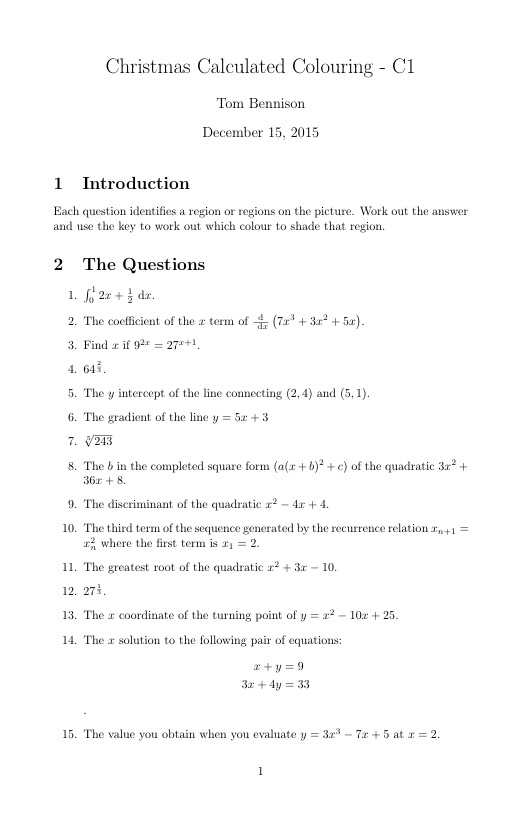I’d love for other people to use this so I have made the sheets available on my website. Please use them in the run up to the end of term.

The picture is available here and the questions here.

Categories

## Some Things to Consider Before Monday’s #mathsjournalclub

Next Monday (that is Monday 7th December) we have our third #mathsjournalclub discussion. We will be chatting about Ellie Darlington’s article “Contrasts in Mathematical Challenges in A-Level Mathematics and Further Mathematics, and Undergraduate Examinations“.

• The findings certainly match my experience of undergraduate mathematics, do they yours? Personally I think closer to 60% of undergraduate exams would be category A.
• Do wee agree that the MATH taxonomy is a useful way to categorise math examination questions?
• What are the limitations of the MATH taxonomy?
• Is this difference between sixth form and undergraduate exams actually a problem?
• There is a suggestion that this taxonomy isn’t much affected by student’s perception of difficulty. Do you think this is true?

I particularly like the table of questions as categorised by the taxonomy: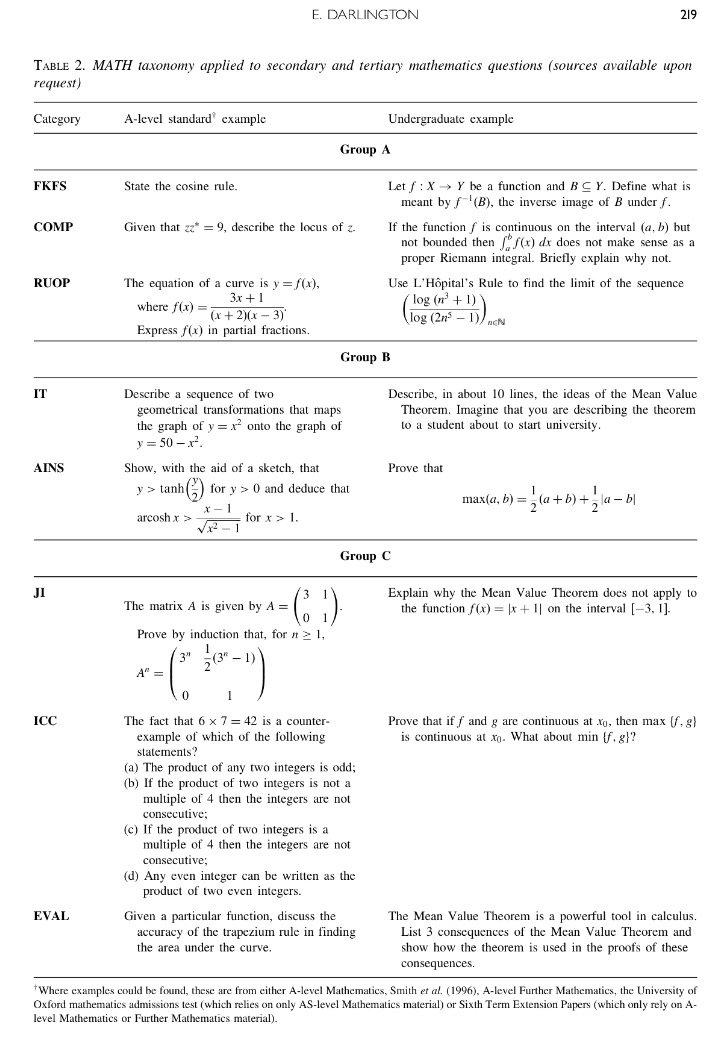I would like to know if the final A-Level standard question is from a real paper as it is unlike any A-Level question I have seen. And I’m also not sure what kind of answer they are looking for as it is actually dependent on the type of integrand (that is, whether you get super-convergence effects).

I’m looking forward to discussions this article on Monday 7th December 2015. I hope you can make it.

Categories

## Something I Struggle to Understand

At school I always found mathematics easy, and fluency with numbers came easy to me, as a consequence I find it hard to understand how students can come to secondary school and not be flent with manipulating the number system, especially the integers.

Earlier this week I asked a KS3 student to tell me how many 20s were in 120. In answer to this they said seven and when I asked how they arrived at this they counted up in the following way: 20,40,60,80,91,102,120. I later went back to them and asked them to count up in 20s and they gave me the same list of numbers. I am struggling to explain this and wondering about the following:

• Why did they count up in this way?
• Is there anything special about the numbers 91 and 102?
• What barriers are there that mean they haven’t developed this number processing fluency?
• How can we develop this?
• Can we tackle this quickly?
• How can we develop the fluency so that the student recognises that 120/20 can simply be calculated by working out 12/2?

I’d love to know your thoughts on these questions, or anything else for that matter.